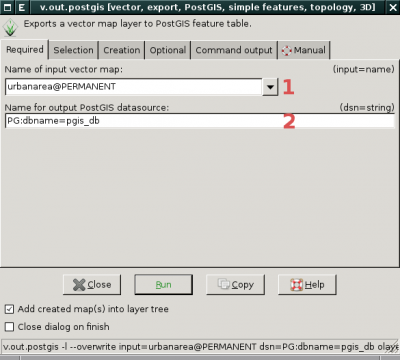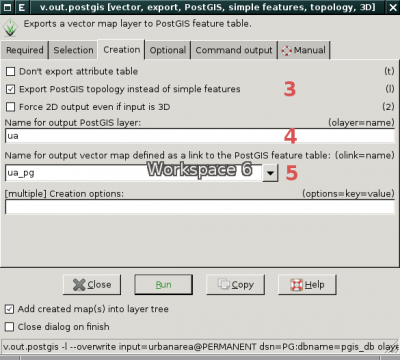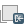# Difference between revisions of "PostGIS Topology"

PostGIS Topology allows to store topological vector data in PostGIS database. It's available in PostGIS 2.0 and later. GRASS GIS supports PostGIS Topology in version GRASS 7.0 and later through GRASS-PostGIS data provider.

Additional info can be found also in the presentation Working with PostGIS Topology in GRASS GIS (Seminar: Advances in GIS data processing and management at Fondazione Edmund Mach, Italy, 2013-04-13).

## Background

Topological models used by GRASS GIS and PostGIS Topology are different - see below.

### GRASS GIS Topology Data Model

GRASS GIS data model defines various topological elements:

• nodes - 0D elements
For each node is defined which lines/boundaries starts and ends in this node.
• lines - 1D elements which cannot form areas
For each line is defined a start and end node.
• boundaries - 1D elements which can form areas
For each boundary is defined a start and end node, and an area on the left and right.
• centroids - point located inside area
For each centroid is defined an area
• areas - 2D elements formed by closed set of boundaries and optionally by one centroid located inside the area
For each area is defined the list of boundaries which forms the area (outer ring), and the list of isles located inside the area.
• isle - 2D elements formed by areas
For each isle is defined the list of boundaries which forms the isle (it's outer ring), and optionally by the area where the isle is located.

For other info and examples, see GRASS GIS programmer's manual.

### PostGIS Topology Model

PostGIS Topology model is based on ISO standard 13249. The model defines only three topological elements:

• nodes - 0D elements
Defined by geometry (point) and by the face where the node is located (can be NULL)
• edges - 1D elements
Defined by geometry (linestring), start and end node, next left and right edge (ie. connectivity) and by the face on the left and right.
• faces - 2D elements
Defined by bounding box.

PostGIS Topology validation: see for example here

### Comparison of GRASS and PostGIS Topology Data Models

GRASS GIS PostGIS Topology
Nodes
nlines,lines,angles containing_face, geom(Point)
Edge
start/end node, next left/right edge, left/right face, geom (LineString)
Line
start/end node
Boundary
start/end node, left/right area
Centroid
area
Face
mbr(Polygon)
Area
nlines,lines,nisles,isle,centroid
Isle
nlines,lines,area

## PostGIS Topology implementation in GRASS GIS

• Points are stored as isolated nodes (containing_face is null)
• Centroids are stored as isolated nodes (containing_face is not null)
• Lines are stored as edges (left_face and right_face is 0)
• Boundaries are stored edges
• Areas are stored as faces (with id > 0)
• Isles are stored as faces (with id <= 0) (including universal face defined by PostGIS Topology)

Additional topological data related to nodes, lines, areas, and isles are stored in separated tables, see figure bellow.

Storing GRASS-related topological data in DB can be disabled by TOPO_GEO_ONLY option, see v.out.postgis for details.

GRASS also stores additional metadata in 'topology.grass' table, currently only bounding box of the map.

```SELECT * from topology.grass;

topology_id |                                      bbox
-------------+---------------------------------------------------------------------------------
...
767 | BOX3D(264003.759398496 55720.5513784461 0,654141.604010025 270194.235588972 0)
...
```

### Example

In GRASS native format:

``` |   Number of points:       0               Number of centroids:  2          |
|   Number of lines:        0               Number of boundaries: 4          |
|   Number of areas:        3               Number of islands:    2          |
```

Topology dump for native GRASS format (v.build):

```Nodes (3 nodes, alive + dead):
node = 1, n_lines = 3, xyz = 100.000000, 0.000000, 0.000000
line =   3, type = 4, angle = 0.000000 (0.0000)
line =   2, type = 4, angle = 1.570796 (90.0000)
line =   1, type = 4, angle = 3.141593 (180.0000)
node = 2, n_lines = 3, xyz = 100.000000, 100.000000, 0.000000
line =  -2, type = 4, angle = -1.570796 (270.0000)
line =  -3, type = 4, angle = 0.000000 (0.0000)
line =  -1, type = 4, angle = 3.141593 (180.0000)
node = 3, n_lines = 2, xyz = 110.000000, 25.000000, 0.000000
line =   6, type = 4, angle = 0.000000 (0.0000)
line =  -6, type = 4, angle = 1.570796 (90.0000)
-----------------------------------
Lines (6 lines, alive + dead):
line = 1, type = 4, offset = 18, n1 = 1, n2 = 2, left = -1, right = 1
line = 2, type = 4, offset = 87, n1 = 1, n2 = 2, left = 1, right = 2
line = 3, type = 4, offset = 124, n1 = 1, n2 = 2, left = 2, right = -1
line = 4, type = 8, offset = 193, area = 1
line = 5, type = 8, offset = 222, area = 2
line = 6, type = 4, offset = 251, n1 = 3, n2 = 3, left = 3, right = -2
-----------------------------------
Areas (3 areas, alive + dead):
area = 1, n_lines = 2, n_isles = 0 centroid = 4
line =   1
line =  -2
area = 2, n_lines = 2, n_isles = 1 centroid = 5
line =   2
line =  -3
isle =   2
area = 3, n_lines = 1, n_isles = 0 centroid = 0
line =  -6
-----------------------------------
Islands (2 islands, alive + dead):
isle = 1, n_lines = 2 area = 0
line =  -1
line =   3
isle = 2, n_lines = 1 area = 2
line =   6
```

In PostGIS Topology model will be this example stored as

• 3 nodes (n1, n2, n3)
```SELECT node_id,containing_face,st_astext(geom) from topo_example.node;

node_id | containing_face |                st_astext
---------+-----------------+------------------------------------------
1 |                 | POINT(452944.862155388 246704.260651629)
2 |                 | POINT(453966.165413534 122105.263157895)
3 |                 | POINT(475413.533834587 128233.082706767)
```
• 4 edges (3, 4, 5, 6)
```SELECT edge_id,next_left_edge,next_right_edge,left_face,right_face,st_geometrytype(geom) from topo_example.edge;
edge_id | next_left_edge | next_right_edge | left_face | right_face | st_geometrytype
---------+----------------+-----------------+-----------+------------+-----------------
1 |             -4 |               3 |         0 |          1 | ST_LineString
2 |              2 |              -2 |         2 |          1 | ST_LineString
3 |             -1 |               4 |         1 |          3 | ST_LineString
4 |             -3 |               1 |         3 |          0 | ST_LineString
```
• 3 faces (two areas and one hole)
```SELECT face_id,st_geometrytype(mbr) from topo_example.face;
face_id | st_geometrytype
---------+-----------------
0 |
1 | ST_Polygon
2 | ST_Polygon
3 | ST_Polygon
```

## How to

### Convert GRASS vector map into PostGIS Topology

Currently GRASS supports converting only selected vector layer into PostGIS table, not the whole vector map (see more about vector layers and maps here).

In the example below GRASS vector map 'urbanareas' (layer '1' which is default) is converted into PostGIS table 'ua' located in 'pgis_db' database using v.out.postgis. Topological export is enabled by `-l` flag. Exported data is linked automatically as GRASS vector map by `olink` parameter. In this case there is no need to create a link using v.external.

From CLI:

```v.out.postgis -l input=urbanarea dsn=PG:dbname=pgis_db olayer=ua olink=ua_pg
```

Important note: Without `-l` flag the vector data are exported as simple features and not as topological elements!

From wxGUI:GUI dialog of v.out.postgis module (required parameters)GUI dialog of v.out.postgis module (other parameters)

### Convert simple features into PostGIS Topology

There are two options.

First option is traditional way which requires converting vector data into GRASS topological format using v.in.ogr and afterwards exporting GRASS topological data into PostGIS Topology by v.out.postgis module. See example below.

```v.in.ogr dsn=zone.shp
```

Second option is based on external data format set by v.external.out. In this case output format is topological PostGIS (see set output vector format to PostGIS Topology)

```v.external.out dsn=PG:dbname=pgis_db options=topology=yes format=PostgreSQL
v.in.ogr dsn=zone.shp
```

In this case, the module v.in.ogr doesn't produce vector data in GRASS format, the data are directly stored in PostGIS Topology. The module also creates vector map given by `olink` parameter which points to the data stored in PostGIS database.

```v.info zone_pg
```
```...
| Map format:      PostGIS (PostgreSQL)                                      |
| DB table:        public.zone                                               |
| DB name:         pgis_db                                                   |
| Geometry column: geom                                                      |
| Feature type:    polygon                                                   |
| Topology:        PostGIS (schema: topo_zone)                               |
| Topology column: topo                                                      |
...
```

See also video tutorials section which contains video showing procedure how to convert simple features (in this case VFK format) into PostGIS Topology in wxGUI.

### Set output vector format to PostGIS Topology

Output format for vector data can be set by v.external.out.

From command line (note `options=topology=yes` which enables topological mode):

```v.external.out output=PG:dbname=pgis_db options=topology=yes format=PostgreSQL
```

Connection settings (username, password, or hostname) can be set by db.login or as a part of connection string (see `dsn` parameter).

From wxGUI menu `File → External formats → Output vector format` or from toolbarAs a result, every newly created vector map will be stored in the given PostGIS Topology database. Created PostGIS data are automatically registered in the current mapset as normal GRASS vector maps (similarly when the user creates link using v.external).

### Create simple features geometry from PostGIS Topology

GRASS can automatically create simple features geometry when building topology over PostGIS Topology features. In example bellow we create buffer map from vector map 'roadsmajor'. The output vector map containing buffers is stored in PostGIS Topology database (`options=topology=yes`). By giving `options=simple_feature=yes` GRASS also stores in DB simple features geometry which is built from PostGIS topological elements (nodes, edges, and faces).

``` v.external.out dsn=PG:dbname=pgis_db options=topology=yes,simple_features=yes format=PostgreSQL
```

We create buffers based on 'roadsmajor' vector map

```v.buffer in=roadsmajor out=roadsmajor_100 dist=100 --o
```

We check result by SQL statement by printing topogeometry data ('topo' column) and area size of created simple features polygons ('geom' column)

```select fid,topo,st_area(geom) from roadsmajor_100;
```
``` fid |    topo     |     st_area
-----+-------------+------------------
1 | (363,1,1,3) | 100002017.849343
```

## Video tutorials

Import VFK data into PostGIS Topology database
Import Shapefile/DXF to PostGIS Topology